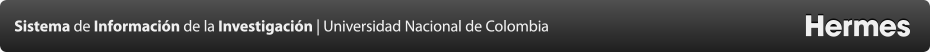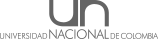Proyectos
A New Empirical Relation between Surface Wave Magnitude and Rupture Length for Turkey Earthquakes
Resumen
Many practical problems encountered in quantitative oriented disciplines entail finding the best approximate solution to an over determined system of linear equations. In this study, it is investigated the usage of different regression methods as a theoretical, practical and correct estimation tool in order to obtain the best empirical relationship between surface wave magnitude and rupture length for Turkey earthquakes. For this purpose, a detailed comparison is made among four different regression norms: (1) Least Squares, (2) Least Sum of Absolute Deviations, (3) Total Least Squares or Orthogonal and, (4) Robust Regressions. In order to assess the quality of the fit in a linear regression and to select the best empirical relationship for data sets, the correlation coefficient as a quite simple and very practicable tool is used. A list of all earthquakes where the surface wave magnitude (Ms) and surface rupture length (L) are available is compiled. In order to estimate the empirical relationships between these parameters for Turkey earthquakes, log-linear fit is used and following equations are derived from different norms: , for L2 Norm regression (R2=0.71), , for L1 Norm regression (R2=0.92), , for Robust regression (R2=0.75), , for Orthogonal regression (R2=0.68), Consequently, the empirical equation given by the Least Sum of Absolute Deviations regression as with a strong correlation coefficient (R2=0.92) can be thought as more suitable and more reliable for Turkey earthquakes. Also, local differences in rupture length for a given magnitude can be interpreted in terms of local variation in geologic and seismic efficiencies. Furthermore, this result suggests that seismic efficiency in a region is dependent on rupture length or magnitude.
Convocatoria
 Nombre de la convocatoria: CONVOCATORIA DEL PROGRAMA NACIONAL PARA LA VISIBILIDAD INTERNACIONAL DE LA PRODUCCIÓN ACADÉMICA MEDIANTE EL APOYO PARA TRADUCCIÓN O CORRECCIÓN DE ESTILO DE ARTÍCULOS DE INVESTIGACIÓN 2013-2015 Modalidad: Modalidad 2. Revisión de estilo de artículos completos, o traducción o revisión de estilo de resúmenes de artículos, que hayan sido aprobados para su publicación e integren un número próximo de revistas de la Universidad Nacional de Colombia indexadas en el IBN-Publindex.
Responsable